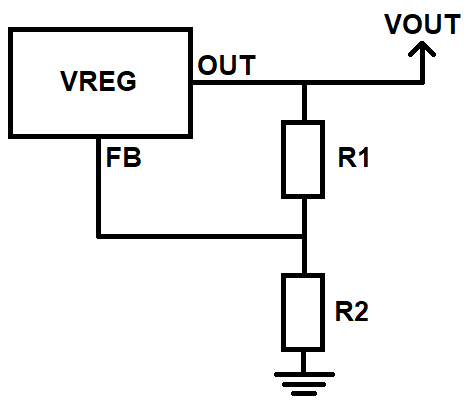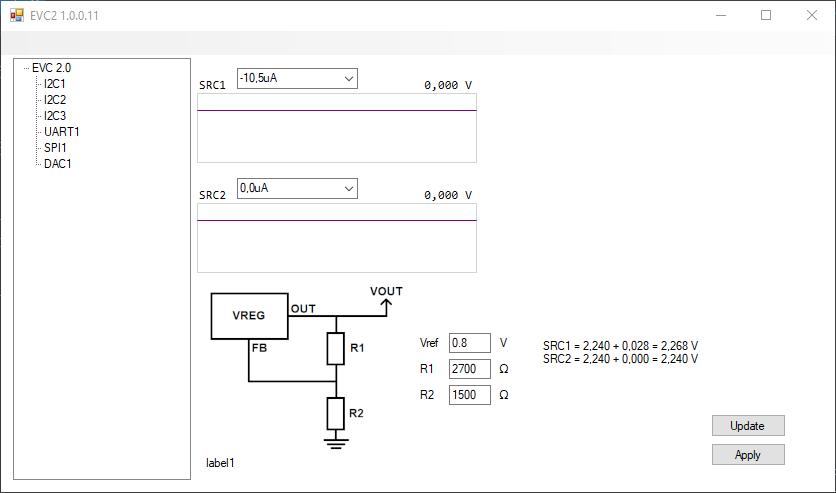Forum breadcrumbs - You are here:
You need to log in to create posts and topics.

# EVC2 VMOD1-header Tutorial

The EVC2 can digitally perform FB (feedback) modification on analog VRM controllers. This works by using an on-board current-DAC which sources or sinks current into the FB pin. The current-DAC is a Maxim DS4404+ and the datasheet has good information on theory of operation: https://datasheets.maximintegrated.com/en/ds/DS4402-DS4404.pdf

• SRCx pins are connected to the current-DAC for sourcing or sinking current from the VRM controller FB pin.
• VINx is for output voltage monitoring. Step-size = 1.6mV, max input 6.6V, tolerant up to 8.0V.
• SRC1 step-size is 2.1μA, range up to +/- 2000μA
• SRC2 step-size is 16.1μA, ranges up to +/- 500μA

Theory of operation

A typical analog VRM controller has a feedback loop with optional compensation network which adjusts the output set-point. Simplified it looks like this:Normally the output voltage is decided by the equation Vout = Vref*(R1/R2 + 1), where Vref is the voltage at the FB pin. This equation and Vref value should be noted in the VRM controller datasheet. Sinking or sourcing current from the FB pin will offset the output by R1*Idac (decrease from sourcing and increase from sinking).

For example the EVC2 on-board LDO (low-dropout regulator) AP7167 (U5 SOP-8L-EP) (https://www.diodes.com/assets/Datasheets/AP7167.pdf) lists this equation and Vref=0.8V. On EVC2 R1 = 2.7kΩ and R2 = 1.5kΩ. You can try yourself using this LDO by soldering SRCx to U5 FB (pin#6) and VINx to U5 OUT (pin#7 or 8). The LDO first needs to be enabled under SPI1 and the output voltage should be set to 2.235V to not have any offset.

Vout = 0.8*(2700/1500+1) = 2.24V

If we sink 10μA (negative) from the FB pin:

Offset = R1*Idac = 2700*10e-6 = 0.027V

Vout+Offset = 2.24V + 0.027V = 2.267V

Practical step by step

1. Measure resistance R1 from FB to Vout
2. Measure resistance R2 from FB to GND
3. Measure Vref voltage at the FB pin
4. Connect SRCx to FB
5. Connect VINx to Vout
6. Enter parameters in the EVC2 software which will help you calculate the resulting voltage
7. First test with sourcing current (positive values) which will decrease the voltage in case the steps are too large